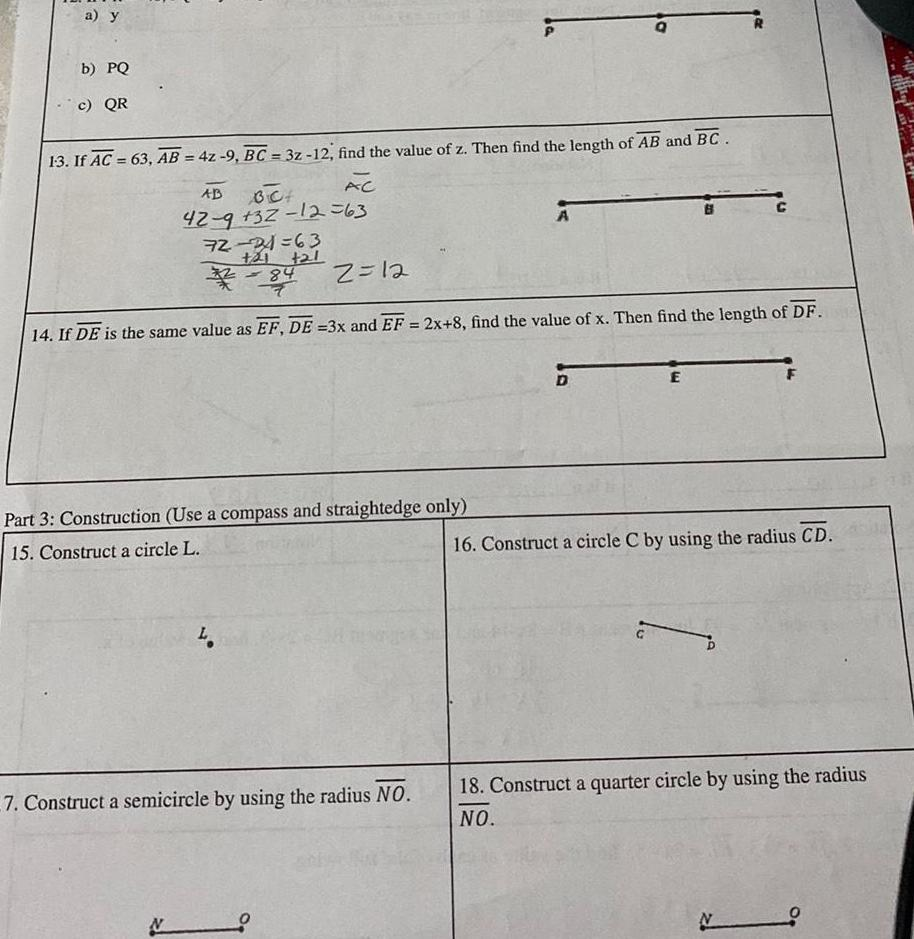Question:

# a y b PQ c QR 13 If AC 63 AB 4z 9 BC 3z 12 find the value of

Last updated: 9/12/2023a y b PQ c QR 13 If AC 63 AB 4z 9 BC 3z 12 find the value of z Then find the length of AB and BC AC AB BC 42 9 32 12 63 72 24 63 21 L N 21 84 L 2 12 14 If DE is the same value as EF DE 3x and EF 2x 8 find the value of x Then find the length of DF Part 3 Construction Use a compass and straightedge only 15 Construct a circle L 7 Construct a semicircle by using the radius NO A D E 16 Construct a circle C by using the radius CD 18 Construct a quarter circle by using the radius NO